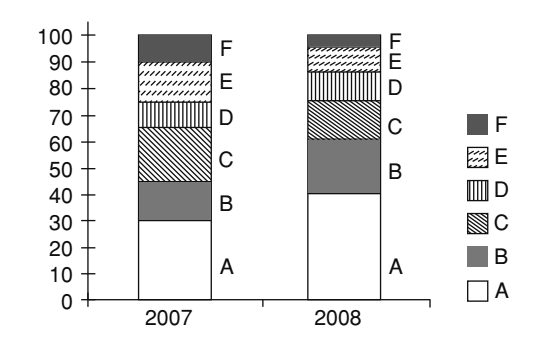Home » Data Interpretation » Bar graph » Question

#### Data Interpretation

Direction: The bar chart given below shows the % distribution of the production of various models of a mobile manufacturing company in 2007 and 2008. The total production in 2007 was 35 Lakh mobile phones and in 2008 the production was 44 Lakhs.
Study the chart and answer the following questions. % of 6 different types of mobiles manufactured by a company over 2 years.1. What was the difference in the number of B type mobiles produced in 2007 and 2008?
1. 3,55,000
2. 2,70,000
3. 2,25,000
4. 1,75,000
##### Correct Option: A

Given:- The total production of mobile phones in 2007 = 35 Lakh
The total production in 2008 = 44 Lakh
Percentage of B type mobiles produced in 2007 = 20%
Percentage of B type mobiles produced in 2008 = 15%
Required difference = The number of B type mobiles produced in 2007 - The number of B type mobiles produced in 2008
Required difference = ( The total production in 2008 x Percentage of B type mobiles produced in 2007 ) - ( The total production of mobile phones in 2007 x Percentage of B type mobiles produced in 2008 )
= 44 x 20% - 35 x 15%

 = 44 x 20 - 35 x 15 100 100

 = 880 - 525 = 355 Million = 355000 100 100